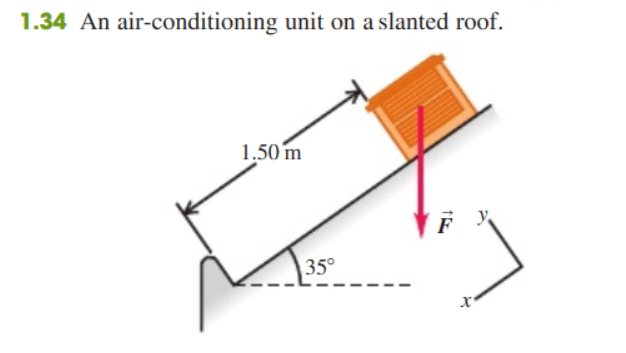# Problem: An air-conditioning unit is fastened to a roof that slopes at an angle of 35° above the horizontal (Fig. 1.34). Its weight is a force F→ on the air conditioner that is directed vertically downward. In order that the unit not crush that roof tiles, the component of the unit's weight perpendicular to the roof cannot exceed 425 N. (One newton, or 1 N, is the SI unit of force. It is equal to 0.2248 lb.)(a) What is the maximum allowed weight of the unit?(b) If the fasteners fail, the unit slides 1.50 m along the roof before it comes to a halt against a ledge. How much work does the weight force do on unit during its slide if the unit has the weight calculated in part a?The work done by a force F→ on the object that undergoes a displacement S→is W=F→·s→.

###### FREE Expert Solution

(a)

From the figure, the magnitude of the weight of the unit acting in the negative y-direction, wy is equal to the normal force.

That is, N = w cosθ

Therefore, w = N/cosθ

89% (469 ratings)###### Problem Details

An air-conditioning unit is fastened to a roof that slopes at an angle of 35° above the horizontal (Fig. 1.34).Its weight is a force $\stackrel{\mathbf{\to }}{\mathbf{F}}$ on the air conditioner that is directed vertically downward. In order that the unit not crush that roof tiles, the component of the unit's weight perpendicular to the roof cannot exceed 425 N. (One newton, or 1 N, is the SI unit of force. It is equal to 0.2248 lb.)

(a) What is the maximum allowed weight of the unit?

(b) If the fasteners fail, the unit slides 1.50 m along the roof before it comes to a halt against a ledge. How much work does the weight force do on unit during its slide if the unit has the weight calculated in part a?

The work done by a force $\stackrel{\mathbf{\to }}{\mathbf{F}}$ on the object that undergoes a displacement $\stackrel{\mathbf{\to }}{\mathbf{S}}$is $\mathbf{W}\mathbf{=}\stackrel{\mathbf{\to }}{\mathbf{F}}\mathbf{·}\stackrel{\mathbf{\to }}{\mathbf{s}}$.